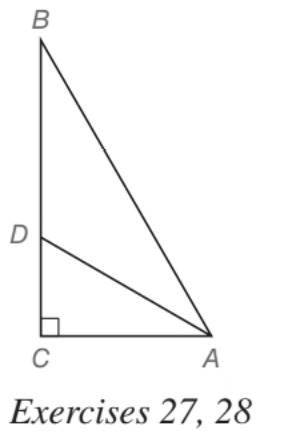Chapter 5.5, Problem 28EElementary Geometry For College St...

7th Edition
Alexander + 2 others
ISBN: 9781337614085

Solutions

Chapter
SectionElementary Geometry For College St...

7th Edition
Alexander + 2 others
ISBN: 9781337614085
Textbook Problem

In Exercises 27 to 33, give both exact solutions and approximate solutions to two decimal places. Given: In ∆ A B C , A D → bisects ∠ B A C A B = 20 and A C = 10 Find: D C and D BTo determine

To find:

DC and DB such that in ABCD, AD bisects BAC, AB=20 and AC=10.

Explanation

Approach:

For a right triangle, for which the measure of the interior angles 30°, 60°, and 90°; if ‘a’ is the length of measure of the shorter leg; opposite to the angle 30°, then the length of the other two sides is given by

Length of the longer leg (opposite to 60°) =a3

Length of the hypotenuse (opposite to 90°)=2a.

In general

Length of the longer leg =3× (Length of the shorter leg)

Length of the hypotenuse =2× (Length of the shorter leg)

Calculation:

Given,

The ABC with bisector of the angle BAC.

AB=20, and

AC=10

Consider the right ABC of the above diagram

Here, AB is the hypotenuse and is of 20 units which is twice the length of the side AC.

But by the theorem of 30-60-90

30°-60°-90° theorem.

In a right triangle whose angle measure 30°, 60°, and 90°, the hypotenuse has a length equal to twice the length of the shorter leg, and the longer leg is the product of 3 and the length of the shorter leg.

we have,

The Hypotenuse = 2 (Length of the shorter side)

Thus, AC is the shorter side of the right triangle ABC and the angle opposite to the measure of the shorter side of a 30°-60°-90° right triangle should be 30°

Thus, ABC=30°.

The third angle of the triangle

BAC=60°

Since, the interior angle A of the triangle ABC is bisected by the ray AD, we have

Thus,

Still sussing out bartleby?

Check out a sample textbook solution.

See a sample solution

The Solution to Your Study Problems

Bartleby provides explanations to thousands of textbook problems written by our experts, many with advanced degrees!

Get Started

43. Solve for This gives a formula for solving two equations in two variables for.

Mathematical Applications for the Management, Life, and Social Sciences

In Exercises 1520, simplify the expression. 18. (2x3) (3x2) (16x1/2)

Applied Calculus for the Managerial, Life, and Social Sciences: A Brief Approach

Evaluate the integral. 18x2/3dx

Single Variable Calculus: Early Transcendentals

Proof Prove that limxcf(x)=L is equivalent to limxc[f(x)L]=0

Calculus: Early Transcendental Functions (MindTap Course List)

True or False: The x-intercepts are the values of x for which f(x) = 0.

Study Guide for Stewart's Single Variable Calculus: Early Transcendentals, 8th

True or False: is conservative.

Study Guide for Stewart's Multivariable Calculus, 8th

In Exercises 116, determine whether the argument is valid. pqqrpr

Finite Mathematics for the Managerial, Life, and Social Sciences